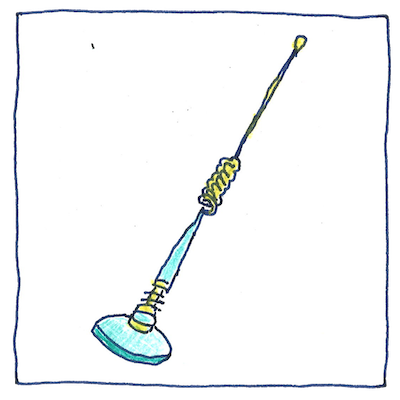1899

The book of science

Tom Sharp

 Oliver Heaviside, George Ashley Campbell, Mihajlo Pupin telecommunication

• Transmissions over transatlantic telegraph cables
• were distorted by an imbalance in the cables
• between capacitive and inductive reactance.
• Oliver Heaviside determined that transmission
• would be free of distortion
• when conductance over capacitance was equal
• to resistance over inductance,
• and suggested balancing the cable
• by increasing its inductance
• Meanwhile,
• aware of the Heaviside condition
• but not of Heaviside’s suggestion
• George Campbell realized
• along the forty-six-mile-long Pittsburgh cable
• at every manhole
• to improve the clarity of transmissions.
• Michael Pupin in the same year
• worked on the problem,
• but loaded his line with capacitors
• rather than inductors. Nevertheless,
• he made a legal claim to Heaviside’s idea,
• are sometimes called Pupin coils.

Heaviside condition

• In a transmission line, when
• the ratio of conductance
• over capacitance
• equals the ratio of resistance
• over inductance,
• when the ratio of siemens per meter
• equals the ratio of ohms per meter
• over henries per meter,
• then a transmission will not be distorted.

Lenz’s law

• Heinrich Friedrich Lenz explained
• that a changing magnetic field in a conductor
• induces a magnetic field in the opposite direction
• which is why in a circuit an inductor
• opposes an alternating current.
• This is called inductive reactance.

Intuitive degree

• At some point, I assume,
• if I study and experiment,
• then the laws of electromagnetism
• will become intuitive. I’ll see
• clearly the relations between
• conductance and resistance,
• capacitance and inductance, and see
• how the tiny components work, but now
• I struggle to comprehend.
• Until then, I’ll feel deprived,
• like a person who knows what is beautiful
• but cannot feel its joy or pain.

An inductor with an inductance of 1 henry produces an electromotive force of 1 volt when the current through the inductor changes at the rate of 1 ampere per second.